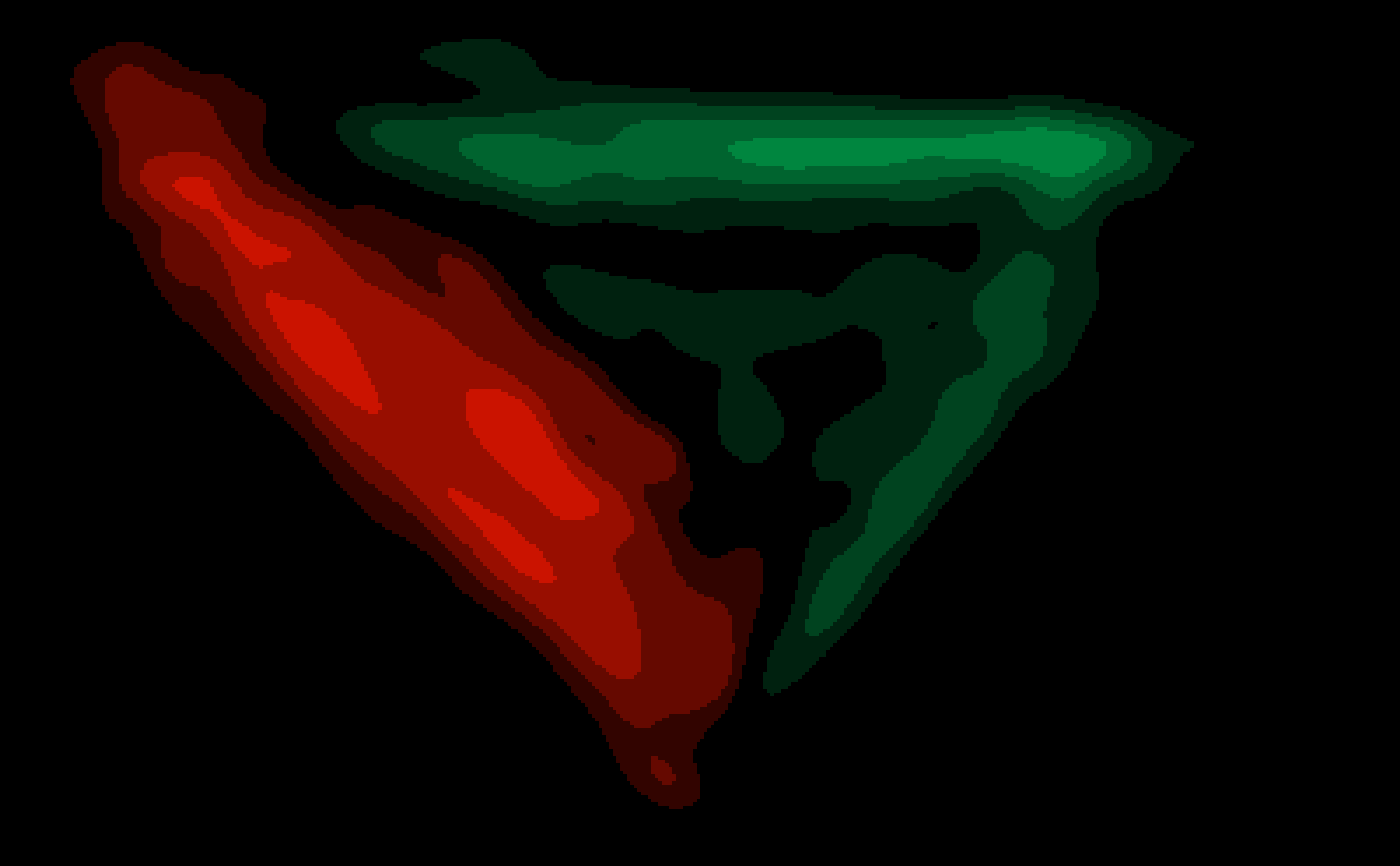mt_diffmap creates a difference-heatmap of the trajectory data using gaussian smoothing. Note that this function has beta status.

mt_diffmap(
x,
y = NULL,
condition = NULL,
use = "trajectories",
dimensions = c("xpos", "ypos"),
use2 = "data",
filename = NULL,
bounds = NULL,
xres = 500,
upscale = 4,
colors = c("#00863F", "#000000", "#FF1900"),
plot = TRUE,
...,
verbose = TRUE
)

## Arguments

x

an object of class mousetrap), a trajectory object of class array, or an object of class mt_heatmap_raw (as created by mt_heatmap_raw).

y

an object of class mousetrap), a trajectory object of class array, or an object of class mt_heatmap_raw (as created by mt_heatmap_raw). The class of y must match the class of x, unless y is NULL.

condition

either a character value specifying which variable codes the two conditions (in x[[use2]]) that should be compared - or a vector matching the number of trajectories in x[[use]] that has exactly two levels. mt_diffmap will create a difference-heatmap comparing all trajectories between the two conditions. If condition is specified, y will be ignored (unless x and y are of class heatmap_raw).

use

a character string specifying which trajectory data should be used.

dimensions

a character vector specifying the trajectory variables used to create the heatmap. The first two entries are used as x and y-coordinates, the third, if provided, will be added as color information.

use2

an optional character string specifying where the data that contain the condition variable can be found. Defaults to "data" as x[["data"]] usually contains all non mouse-tracking trial data.

filename

a character string giving the name of the file. If NULL (the default), the R standard device is used for plotting. Otherwise, the plotting device is inferred from the file extension. Only supports devices tiff, png, pdf.

bounds

numeric vector specifying the corners (xmin, ymin, xmax, ymax) of the plot region. By default (bounds = NULL), bounds are determined based on the data input.

xres

an integer specifying the number of pixels along the x-dimension. An xres of 1000 implies an 1000*N px, where N is determined so that the trajectories aspect ratio is preserved (provided the bounds are unchanged).

upscale

a numeric value by which the output resolution of the image is increased or decreased. Only applies if device is one of tiff, png, or pdf.

a numeric value specifying the standard deviation of the gaussian smoothing. If zero, smoothing is omitted.

colors

a character vector specifying the colors used to color cases of image1 > image2, image1 ~ image2, image1 < image2, respectively. Note that the colors are used in that specific order. Defaults to c("#00863F", "#FFFFFF", "#FF1900") which specifies a green-black-red color gradient.

integer specifying the number of shades for the color gradient between the first and second, and the second and third color in colors.

plot

logical specifying whether resulting image should be plotted (plot = TRUE, the default). If (plot = FALSE), an object of class mt_object_raw is returned.

...

arguments passed to mt_heatmap_raw.

verbose

logical indicating whether function should report its progress.

## Details

mt_diffmap takes two objects that either contain trajectory heatmaps or from which trajectory heatmaps can be computed. Difference-heatmaps are constructed analogously to mt_heatmap_raw.

mt_heatmap and mt_heatmap_ggplot for plotting trajectory heatmaps.

## Author

Dirk U. Wulff

Pascal J. Kieslich

## Examples

mt_diffmap(KH2017, condition="Condition",